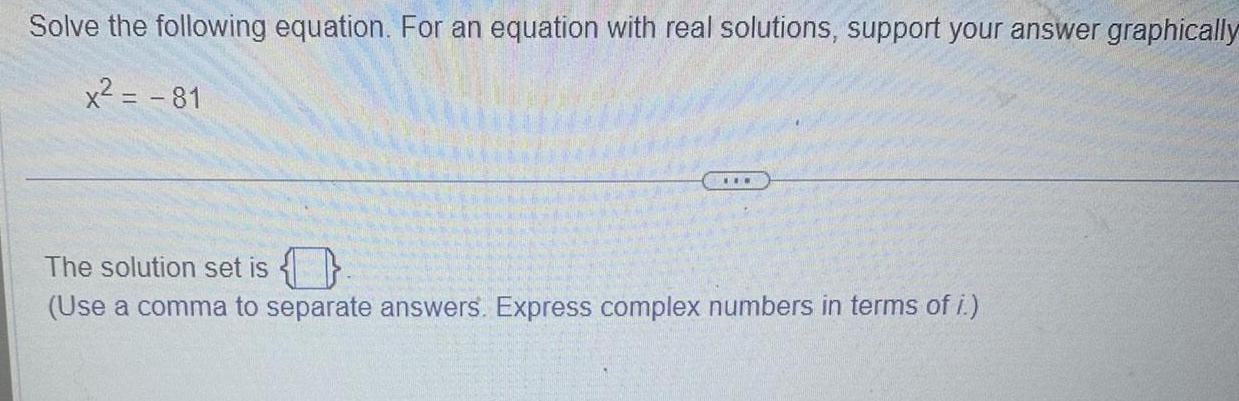Question:

# Solve the following equation For an equation with real

Last updated: 9/19/2023Solve the following equation For an equation with real solutions support your answer graphically x 81 The solution set is Use a comma to separate answers Express complex numbers in terms of i